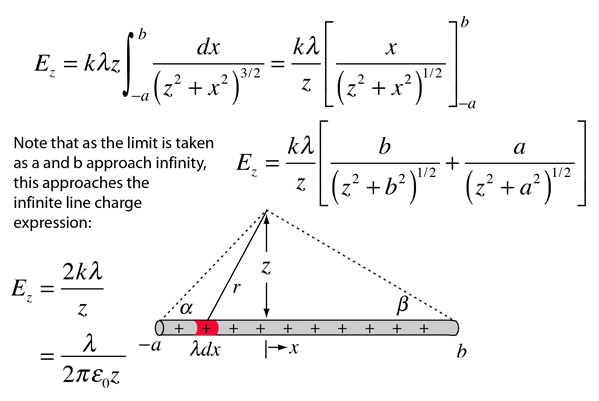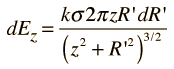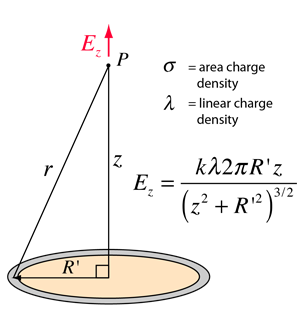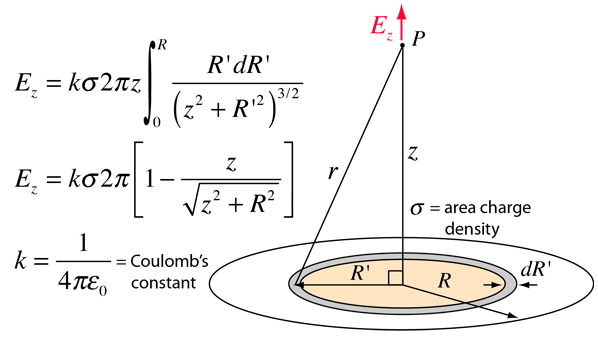# Electric Field of Line Charge

The electric field of a line of charge can be found by superposing the point charge fields of infinitesmal charge elements. The radial part of the field from a charge element is given byThe integral required to obtain the field expression isInfinite line charge
 Electric potential of finite line charge
 Fields for other charge geometries
Index

Electric field concepts

 HyperPhysics***** Electricity and Magnetism R Nave
Go Back

# Electric Field: Ring of Charge

The electric field of a ring of charge on the axis of the ring can be found by superposing the point charge fields of infinitesmal charge elements. The ring field can then be used as an element to calculate the electric field of a charged disc.
 The electric fields in the xy plane cancel by symmetry, and the z-components from charge elements can be simply added.If the charge is characterized by an area density and the ring by an incremental width dR' , then:This is a suitable element for the calculation of the electric field of a charged disc.Electric potential of a ring of charge
 Fields for other charge geometries
Index

Electric field concepts

 HyperPhysics***** Electricity and Magnetism R Nave
Go Back

# Electric Field:Disc of Charge

The electric field of a disc of charge can be found by superposing the point charge fields of infinitesmal charge elements. This can be facilitated by summing the fields of charged rings. The integral over the charged disc takes the formElectric potential of disc of charge
 Fields for other charge geometries
Index

Electric field concepts

 HyperPhysics***** Electricity and Magnetism R Nave
Go Back